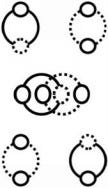# The Most Basic ‘String’ (Vibration) (Fluctuation) (Wave)

If zero, then one. (Meaning, if one, then, zero).Photo by Juan Sisinni on Unsplash

In mathematics, in ‘string’ theory, we have an open and a closed ‘string.’

Which is an ‘other’ way of saying we have a ‘zero’ and a ‘one.’

So, an intelligent observer should ask, where does the ‘zero’ and ‘one’ come from?

If zero, then one, means, if one, then zero. Which defines the most basic ‘string.’ (Neither zero nor one; both zero and one; zero and-or one; zero is one.)

This is proven, easily, by the abstraction: if circumference then diameter (meaning if diameter then circumference). This gives us the basis for ‘conditionality,’ and ‘similarity,’ explaining how ancient people were able to ‘build the pyramids.’If zero, then one (if one, then zero).

That is, it’s basic math, which leads to basic physics, that the most basic string, is a self-referential recursion (quantum entanglement) called (the conservation of) a(n) (uber-basic) circle.

Explaining fluctuations, vibrations, and waves. Sometimes thought of as ‘quantum foam.’

Meaning, the circular relationship between circumference and diameter, produces the linear relationship between diameter and circumference. Explaining both repetition (reproduction) and sequence (reproduction). Basic ‘movement.’ Motion, in general. The (circular) (linear) relationship between general and specific (any X and Y).Reproduction (General and Specific) (Repetition and Sequence)

Where zero is half-the-time one. Meaning zero is all-the-time one. Because it is impossible to have a half without a whole. A zero without a one. A circle without a line.

Conservation of the Circle explains the circular relationship between ‘beginning’ and ‘end.’ Before and after. Open and closed. Zero and one.